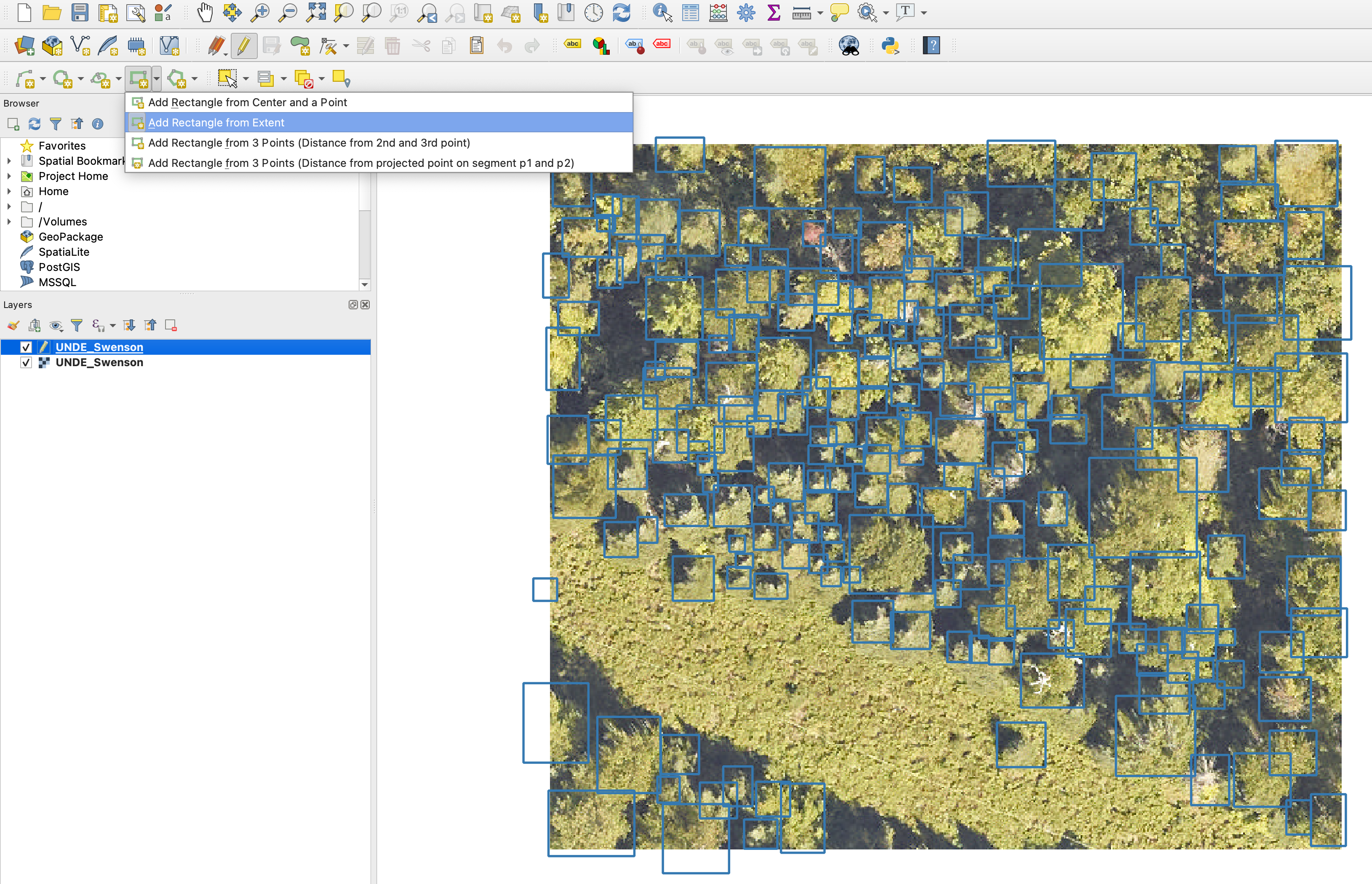# Annotation#

Annotation is likely the most important part of machine learning projects. Fancy models are nice, but data is always paramount. If you aren’t happy with model performance, annotating new samples is always the best first idea.

## How should I annotate images?#

For quick annotations of a few images, we recommend using QGIS or ArcGIS. Either as project or unprojected data. Create a shapefile for each image.## Do I need annotate all objects in my image?#

Yes! Object detection models use the non-annotated areas of an image as negative data. We know that it can be exceptionally hard to annotate all trees in an image, or determine the classes of all birds in an image. However, if you have objects in the image that are not annotated, the model is learning to ignore those portion of the image. This can severely affect model performance.

## Can I annotate points instead of bounding boxes?#

Yes. This makes more sense for the bird detection task, as trees tend to vary widely in size. Often, birds will be a standard size compared to the image resolution.

If you would like to train a model, here is a quick video on a simple way to annotate images.

Using a shapefile, we could turn it into a dataframe of bounding box annotations by converting the points into boxes. If you already have boxes, you can exclude convert_to_boxes and buffer_size.

```df = shapefile_to_annotations(
shapefile="annotations.shp",
rgb="image_path", convert_to_boxes=True, buffer_size=0.15
)
```

Optionally, we can split these annotations into crops if the image is large and will not fit into memory. This is often the case.

```df.to_csv("full_annotations.csv",index=False)
annotations = preprocess.split_raster(
path_to_raster=image_path,
annotations_file="full_annotations.csv",
patch_size=450,
patch_overlap=0,
base_dir=directory_to_save_crops,
allow_empty=False
)
```

## How can I view current predictions as shapefiles?#

It is often useful to train new training annotations starting from current predictions. This allows users to more quickly find and correct errors. The following example shows how to create a list of files, predict detections in each, and save as shapefiles. A user can then edit these shapefiles in a program like QGIS.

```from deepforest import main
from deepforest.visualize import plot_predictions
from deepforest.utilities import boxes_to_shapefile

import rasterio as rio
import geopandas as gpd
from glob import glob
import os
import matplotlib.pyplot as plt
import numpy as np
from shapely import geometry

files = glob("{}/*.JPG".format(PATH_TO_DIR))
m = main.deepforest(label_dict={"Bird":0})
m.use_bird_release()
for path in files:
#use predict_tile if each object is a orthomosaic
boxes = m.predict_image(path=path)
#Open each file and get the geospatial information to convert output into a shapefile
rio_src = rio.open(path)

#Skip empty images
if boxes is None:
continue

#View result
image = np.rollaxis(image, 0, 3)
fig = plot_predictions(df=boxes, image=image)
plt.imshow(fig)

#Create a shapefile, in this case img data was unprojected
shp = boxes_to_shapefile(boxes, root_dir=PATH_TO_DIR, projected=False)

#Get name of image and save a .shp in the same folder
basename = os.path.splitext(os.path.basename(path))
shp.to_file("{}/{}.shp".format(PATH_TO_DIR,basename))
```